# Statement of Parallel Axis Theorem: Notes pdf ppt Engineering Mechanics

## Parallel Axis Theorem:

The theorem determines the moment of inertia of a rigid body about any given axis, given that moment of inertia about the parallel axis through the center of mass of an object and the perpendicular distance between the axes.

## Statement:

The moment of inertia about Z-axis can be represented as: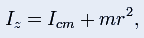Where
Icmis the moment of inertia of an object about its centre of mass
m is the mass of an object
r is the perpendicular distance between the two axes.

## Proof:

Assume that the perpendicular distance between the axes lies along the x-axis and the centre of mass lies at the origin.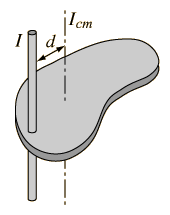The moment of inertia relative to z-axis that passes through the centre of mass, is represented as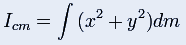Moment of inertia relative to the new axis with its perpendicular distance r along the x-axis, is represented as: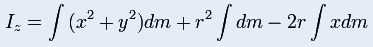We get,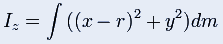The first term is Icm,the second term is mr2and the final term is zero as the origin lies at the centre of mass. Finally,

## For Area Moments of Inertia: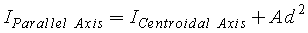: is the cross-sectional area.: is the perpendicuar distance between the centroidal axis and the parallel axis.

## For Area Radius of Gyration: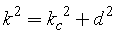: is the Radius of Gyration about an axis Parallel to the Centroidal axis.: is the Radius of Gyration about the Centroidal axis.: is the perpendicuar distance between the centroidal axis and the parallel axis.

## For Mass Moments of Inertia: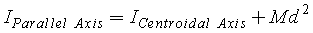: is the mass of the body.: is the perpendicuar distance between the centroidal axis and the parallel axis.

## For Mass Radius of Gyration:: is the Radius of Gyration about an axis Parallel to the Centroidal axis.: is the Radius of Gyration about the Centroidal axis.: is the perpendicuar distance between the centroidal axis and the parallel axis.

### Feedback is important to us.

error: Content is protected !!